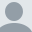# ColdFusion Spreadsheet Bug with Formulas

This post is more than 2 years old.

A few days ago a reader asked me how to use ColdFusion to read the value of a spreadsheet cell that was defined by a value. I quickly created a spreadsheet where I used a sum formula to give the total of a number in the first two columns. ColdFusion allows you then to get both the actual value and the formula which is kinda cool. Consider:

`<cfset f = expandPath("./book1.xlsx")> <cfset s = spreadsheetRead(f)> <cfset value = spreadsheetGetCellValue(s, 1,3)> <cfoutput>Value in C1 is ...#value#</cfoutput> <p> <cfset formula = spreadsheetGetCellFormula(s, 1,3)> <cfoutput>Based on formula... #formula#</cfoutput> <p>`

What's cool is that the spreadsheet object is "live" - if you change the value of one one of the numbers and run spreadsheetGetCellValue again, you will see the updated result. The reader took my code and tried it on his spreadsheet but something weird happened. The exact same code (except for what row/cell he used) returns the formula for both calls! I thought perhaps it was a file issue. But when I replaced his formula with a simple Sum it worked correctly again. I have no idea why his formula 'broke' ColdFusion's getCellValue call. He has already logged a bug report for it. For those who are curious, here is the formula. It's not simple - but it seems like it should be work (note - I added a few spaces to make it wrap nicely):

=(IF(\$B\$3>=\$B16,0, MIN(((1-\$B\$7)\$B\$6((1+\$B\$11)^(((YEAR(EOMONTH(\$B16,0))- YEAR(EOMONTH(\$B\$3,0)))12)+ MONTH(EOMONTH(\$B16,0))- MONTH(EOMONTH(\$B\$3,0)))-1)),\$B\$8))- IF(\$B\$3>=\$B16,0,MIN(((1-\$B\$7)\$B\$6* ((1+\$B\$11)^(((YEAR(EOMONTH(\$B16,-1))- YEAR(EOMONTH(\$B\$3,0)))12)+ MONTH(EOMONTH(\$B16,-1))-MONTH(EOMONTH(\$B\$3,0)))-1)),\$B\$8))) (1/DAY(EOMONTH(B16,0)))

Raymond Camden's Picture

## About Raymond Camden

Raymond is a senior developer evangelist for Adobe. He focuses on document services, JavaScript, and enterprise cat demos. If you like this article, please consider visiting my Amazon Wishlist or donating via PayPal to show your support. You can even buy me a coffee!

Lafayette, LA

### Archived CommentsComment 1 by Dan G. Switzer, II posted on 6/3/2011 at 10:24 PM

My guess would be either (1) he's using a function in the formula that's not excepted or (2) the formula is throwing an exception in the one of the Java libraries and just returning the formula when the exception occurs. The formula's complex enough that it would be easy to have an error in their somewhere--even if the formula is technically correct, some of the data in the cells may be causing an issue.Comment 2 by Raymond Camden posted on 6/3/2011 at 10:38 PM

But when viewed in Excel, his formula works fine.Comment 3 by Brian posted on 6/4/2011 at 12:51 AM

FYI: tried that formula in an xls with Nadel's POI on CF8, was able to pull both the data value and the formula without a problem. As I obviously had no valid data in the referneced cells, I got a '0' for the value (which is what the spreadsheet displayed) and the formula for the GetCellFormula call.Comment 4 by Raymond Camden posted on 6/4/2011 at 12:57 AM

Thanks for testing that Brian. I pinged the original reader to see if he can try too.Comment 5 by John Gerald posted on 6/4/2011 at 7:33 PM

Just tried Ben Nadel's POI using Coldfuion 9 and it works like a charm! Thanks Raymond for posting this and thanks to both Dan and Brian for your input.Comment 6 by Mark posted on 6/7/2011 at 5:35 AM

I don't have a solution but an explanation of why it wont show the value for the formula using spreadsheetGetCellValue. After some testing I can confirm that spreadsheetGetCellValue does not support addins/user made functions.

EOMONTH in older version of Excel was part of analysis tool pack and not built into the base excel formulas. While in the newest excel version you can use it without the analyst toolpack my guess is spreadsheetGetCellValue still don't recognized it as a excel function.

If you remove all references to EOMONTH from formula it will work as expected.Comment 7 by Raymond Camden posted on 6/7/2011 at 5:37 AM

Wow Mark. Thank you.Comment 8 by Howard Fore posted on 7/14/2011 at 10:04 PM

Not so fast. I'm getting the formula instead of a value where there's no custom anything in the formula: PERCENTILE(C\$14:C\$134,0.9)Comment 9 by Raymond Camden posted on 7/24/2011 at 10:54 PM

Well crap then. :\Comment 10 by Dave Phillips posted on 11/21/2012 at 9:06 PM

Does anyone know if this bug is fixed in ColdFusion 10 ?